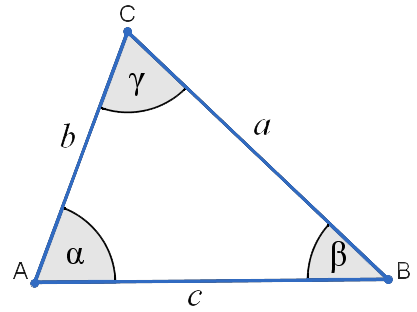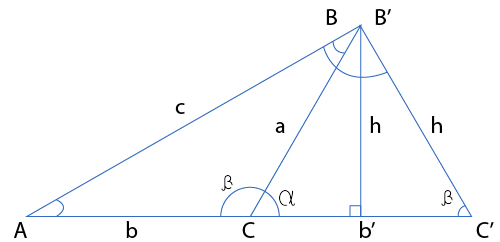Uh Oh! It seems you’re using an Ad blocker!

Since we’ve struggled a lot to makes online calculations for you, we are appealing to you to grant us by disabling the Ad blocker for this domain.# Law of Sines CalculatorCalculate:

$$A = \sin^{-1} \left[ \dfrac{a \sin B}{b} \right]$$

$$A = \sin^{-1} \left[ \dfrac{a \sin C}{c} \right]$$

$$B = \sin^{-1} \left[ \dfrac{b \sin A}{a} \right]$$

$$B = \sin^{-1} \left[ \dfrac{b \sin C}{c} \right]$$

$$C = \sin^{-1} \left[ \dfrac{c \sin A}{a} \right]$$

$$C = \sin^{-1} \left[ \dfrac{c \sin B}{b} \right]$$

$$a = \dfrac{b \sin A}{\sin B}$$

$$a = \dfrac{c \sin A}{\sin C}$$

$$b = \dfrac{a \sin B}{\sin A}$$

$$b = \dfrac{c \sin B}{\sin C}$$

$$c = \dfrac{a \sin C}{\sin A}$$

$$c = \dfrac{b \sin C}{\sin B}$$

Side a

Side b

Side c

Angle A (α)

Angle B (β)

Angle C (γ)

Table of Content
 1 Equations Derived from Law of Sines for Angles A, B, and C: 2 Derived Equations from Law of Sines Solving for Sides a, b, and c: 3 Ambiguous Case Law of Sines: 4 When to use the Law of Sines? 5 What is the Main Rule for the Sides of a Triangle? 6 What is Oblique Triangle in Trigonometry? 7 What are the Characteristics of a Triangle?
Get The Widget!

Add Law of Sines Calculator to your website to get the ease of using this calculator directly. Feel hassle-free to account this widget as it is 100% free, simple to use, and you can add it on multiple online platforms.

An online law of sines calculator allows you to find the unknown angles and lengths of sides of a triangle. When we dealing with simple and complex trigonometry sin(x) functions, this calculator uses the law of sines formula that helps to find missing sides and angles of a triangle.

## What is the Law of Sines?

The Laws of sines are the relationship between the angles and sides of a triangle which is defined as the ratio of the length of the side of a triangle to the sine of the opposite angle.Where:

Sides of Triangle:

$$a = side a, b = side b, c = side c$$

Angles of Triangle:

$$A = angle A, B = angle B, C = angle C$$

Characteristics of Triangle:

P = Triangle perimeter, s = semi-perimeter, K = area, r = radius of inscribed circle, R = radius of circumscribed circle

If a, b, and c are the length of sides of a triangle and opposite angles are A, B, and C respectively; then law of sins shows:

$$a/sinA = b/sinB = c/sinC$$

So, the law of sine calculator can be used to find various angles and sides of a triangle.

Example:

Compute the length of sides b and c of the triangle shown below.Solution:
Here, calculate the length of the sides, therefore, use the law of sines in the form of
$$\frac{a}{sin A} = \frac{b}{sin B}$$
Now,
$$\frac{a}{sin 100^0}= \frac{12}{sin 50^0}$$
By Cross multiply:
$$12 sin 100^0= a sin 50^0$$
Both sides divide by sin $$50^0$$
$$a = \frac{(12 sin 100^0)}{sin 50^0}$$
From the calculator we get:
$$a = 15.427$$
So, the length of sides b and c is $$15.427 mm$$.

However, an Online Sine Calculator will calculate the sine trigonometric function for the given angle in degree, radian, or the π radians.

### Equations Derived from Law of Sines for Angles A, B, and C:

These are some equations that are used by the law of sines calculator which are obtained from the law of sins:

$$A=sin^{−1}[\frac{asinB}{b}]$$

$$A=sin^{−1}[\frac{asinC}{c}]$$

$$B=sin^{−1}[\frac{bsinA}{a}]$$

$$B=sin^{−1}[\frac{bsinC}{c}]$$

$$C=sin^{−1}[\frac{csinA}{a}]$$

$$C=sin^{−1}[\frac{csinB}{b}]$$

### Derived Equations from Law of Sines Solving for Sides a, b, and c:

In order to find any side of a triangle law of sines calculator fetched some values from law of sines formula:

$$a=\frac{bsinA}{sinB}$$

$$a=\frac{csinA}{sinC}$$

$$b=\frac{asinB}{sinA}$$

$$b=\frac{csinB}{sinC}$$

$$c=\frac{asinC}{sinA}$$

$$c=\frac{bsinC}{sinB}$$

Also, you can find alpha (α) by using, $$a=n/a, b=n/a, beta (β ) =n/a$$ values, while the value of beta is calculated by using $$a=n/a, alpha=n/a, b=n/a$$.

## Ambiguous Case Law of Sines:

An ambiguous case occurs, when two different triangles constructed from given data then the triangles are $$ABC \text{ and} AB’C’$$.There are some conditions to use the law of sines for the case to be ambiguous:

• When only sin(a)sin(b) and an angle A given.
• The angle of A is less than $$90^0$$.
• Side a is shorter as compared to side c.
• Side a is longer than altitude h from the angle B where a > h.

Furthermore, The online CSC Calculator will determine the cosecant and sin inverse trigonometric function for the given angle it either in degree, radian, or the pi (π) radians.

## How Law of Sines Calculator Works?

The law of sine calculator especially used to solve sine law related missing triangle values by following steps:

### Input:

• You have to choose an option to find any angle or side of a trinagle from the drop-down list, even the calculator display the equation for the selected option
• Now, you need to add the value for angles and sides into the designated fields
• Then, you have to select the units for the entered values
• At last, make a click on the given calculate button

### Output:

The law of sines calculator calculates:

• The value of angles and sides for the given equation
• The values for the different characteristics of  a triangle
• Diagram

## FAQ’s

### When to use the Law of Sines?

When you have two sides and one angle or two angles and one side of a triangle then we use laws of sines.

### What is the Main Rule for the Sides of a Triangle?

According to the triangle inequality theorem, the sum of any two sides must be greater than the third side of a triangle and this rule must fulfil all three conditions of sides.

### What is Oblique Triangle in Trigonometry?

An oblique triangle is not a right triangle so basic trigonometric ratios do not apply, they can be modified to cover oblique by using sines and cosines law.

### What are the Characteristics of a Triangle?

There are different ways to find triangle characteristics:

• Radius of circle around triangle $$R = (abc) / (4K)$$
• Radius of inscribed circle in a triangle $$r = \sqrt{(s-a)*(s-b)*(s-c) / s}$$
• Triangle area $$K = \sqrt{ s*(s-a)*(s-b)*(s-c)}$$
• Triangle semi perimeter $$s = 0.5 * (a + b + c)$$
• Perimeter $$P = a + b + c$$

## End-Note:

The law of sines calculator is highly recommendable for assessing the missing values of a triangle by using the law of sines formula. Finding all these values manually is a difficult task, also it increases the risk to get accurate results. By using the law of sine calculator you can find all sine law values instantly and 100% error-free. Moreover, this tool is beneficial for people who work with the law of sine related trigonometric function.

## Reference:

From the source of Wikipedia: The ambiguous case of triangle solution, Relation to the circumcircle, Relationship to the area of the triangle.

From the source of Dave’s Short Trig Course: Oblique Triangles, Pythagorean theorem, Triangle Characteristics.

From the source of Khan Academy: Laws of sines and cosines review, Solving triangles using the law of sines, Missing Angle.